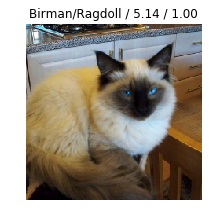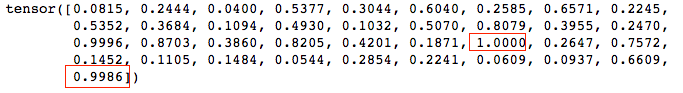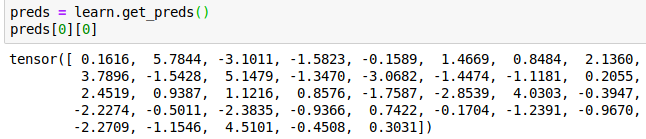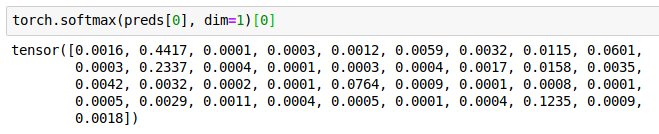# Understanding why plot_top_losses shows a probability of 1.00 for the class the model got wrong

Hi everyone !

During the lecture I struggled to understand why the function plot_top_losses, which outputs the top errors the model made with the image and some more info, showed a probability of 1.00 for the right class for some of the images the model got wrong. If the model got it wrong, it shouldn’t give the right class a probability of 1.00, right ?

With the help of @KevinB and a dive into the source code I think I finally understood what’s happening. Hopefully this will help someone else.

Here’s an example of output of the plot_top_losses functionIn this example, the model thought this was a Birman cat when in fact it was a Ragdoll cat. The loss on this data was of 5.14, and the probability of the Ragdoll class was… 1.00. Weird right ?

Well, here’s all the predictions for that example :In red are the two interesting predictions (and keep in mind, these aren’t really probabilities and we can’t even think of them as such, as they don’t add up to 1 – they are only numbers the model output for each class, the higher the more probable the class is) :

• 1.000 is the prediction for the Birman class (index 24). It’s the highest so that’s what the model predicted.
• 0.9986 is the prediction for the real class (Ragdoll). We can see it’s really close to 1.

Now let’s look at what plot_top_losses is showing :

``````f'{classes[self.pred_class[idx]]}/{classes[t]} / {self.losses[idx]:.2f} /
{self.probs[idx][t]:.2f}'
``````

Aha ! So the last number is only showed with a precision of 2 decimal point (as indicated by .2f). Hence why 0.9986 is displayed as being 1.00 even though it’s not the highest prediction.

Voila, I hope I was clear and this will help someone !

A suggestion for the fast.ai dev team : maybe it would be clearer if plot_top_losses output the full decimals of the prediction ?

28 Likes

Excellent discussion and analysis. What this actually shows is that I failed to use softmax correctly. Oops! Will look at debugging tomorrow. Feel free to fix and send a PR in the meantime if anyone wants to4 Likes

Thank you for your reply ! I’m afraid I’m still a beginner both in deep learning, fast.ai and contributing to open source projects, so I’ll have to leave that to someone elseWow well in that guess I’m doubly impressed. Your analysis was really excellent. I suspect you may be underestimating yourself!

3 Likes

Should the convlearner model itself have a softmax at the end after the linear layer or just the ClassificationInterpretation?

Thank you very much for your kind wordsGreat question! No it shouldn’t, because we’re using a loss function that incorporates the activation function too.

Hmmm… I’m not even sure how best to fix this. Paging @sgugger!

Perhaps the Learner class needs to know about what final activation function to use. Or perhaps we shouldn’t be using loss functions that incorporate the activation function, but instead keep them separate so that predictions always make sense…

It’s more stable to have the loss function with the last activation, so we sould keep it that way. What we can do is have the learner map this loss_fn to a final_activation (we create a private dictionary for this) so that the predictions make more sense.

It should be possible to get this working with:

preds = learn.get_preds()
interp = >ClassificationInterpretation(learn.data,F.softmax(preds,1),preds,loss_class=nn.CrossEntropyLoss,sigmoid=False)

Currently the probabilities seem to be using sigmoid, instead of softmax, so we can add the probabilities for softmax ourselves. Note, the “probability” seems to be that of the True case, not the predicted class. So expect numbers very close to 0 for something that softmax did not choose.

I’m still confused. I don’t know how the algorithm for the output works. It the model got the class wrong, then what should have been the number it outputed for the class? I don’t see anything wrong with the output being 1.

Softmax by definition adds up to 1, so you can’t have multiple numbers being close to one.

Basically the model outputs a 1-dimensionnal tensor (so just an array) of numbers between 0 and 1, one number for each class. Here there are 37 classes (number of breeds of cats and dogs), so the tensor is of size 1x37. For each number in this tensor, the higher the number the more probable it is the image is of the class represented by that number. For example, the 24th number represents the Birman class and in the above example that image had a prediction of 1.0000 for this class. For the prediction, the model then chooses the class with the highest number (meaning the highest probability of being right according to the model).

The ouput of 1.00 (for the actual class, that the model got wrong) in plot_top_losses was weird to me because the predictions are capped by 1. So if the right class was 1.00, it should have choosen this one for the prediction but it didn’t. That’s because is wasn’t really 1.00, it was 1 minus a small number (0.9986 in the example I gave in my post) that got rounded up to 1.00.

And what those guys are saying is that the softmax function should be used to have the sum of the values of the tensor to be 1, so that those numbers would be heuristically closer to probabilities than what they are now – thus way more intuitive.

6 Likes

I’ve moved this to the ‘advanced’ subcategory FYI.

So picking 1 was the best possible answer the algorithm could have given. To make a better prediction, it would have needed to learn or train with more pictures of Ragdoll breeds so it would have been able to tell the difference between the two. The second class could have been 0.9986 or 0.0001. It would have still chosen the highest number. Is that how the plot_top_losses function works?

Yes indeed ! Only the highest number is taken for the prediction, the 36 other numbers are not used at all. However we can look at those numbers to see how close the algorithm was to pick one class over another (for example if it picked the class at 1.0000 but the right one was at 0.9986, then the algorithm was quite close to choose the right one, at least more so than if the right one was way lower).

1 Like

I ran the numbers in the tensors into a softmax function and this is what I got:

array([0.01868258, 0.02198788, 0.01792312, 0.02948234, 0.02334754,
0.03150328, 0.02230011, 0.03322131, 0.02155465, 0.02940873,
0.02489063, 0.01921116, 0.0281935 , 0.01909242, 0.02859099,
0.03862855, 0.02557439, 0.02204513, 0.04679103, 0.04111576,
0.02533259, 0.03911835, 0.02621133, 0.0207634 , 0.04680975,
0.0224388 , 0.0367189 , 0.01991138, 0.01923231, 0.0199752 ,
0.01818308, 0.02290812, 0.02154603, 0.01830166, 0.0189119 ,
0.03334779, 0.04674427])

Heres the original and the softmax version of the particular numbers of interest:

x = 1.000, y = 0.04680975415203642
x[-1] = 0.9986, y[-1] = 0.046744266348382475

2 Likes

Hmm I think Softmax was actually used correctly, maybe @sgugger could have a closer look at this:

In the lesson1 notebook the output of `learn.loss_func:`

• `function torch.nn.functional.cross_entropy [...]`

pytorch docs state:

This criterion combines `log_softmax` and `nll_loss` in a single function.

So the actual model/lossfunc seems correct.

The `ClassificationInterpretation.from_learner(learn)` also correctly has ‘CrossEntropyLoss’(default param), but implicitly sets sigmoid=True (default param) .

Can’t find this in the docs but the source says this:

`self.probs = y_pred.sigmoid() if sigmoid else y_pred`

strangely, looking at the output from learn.get_preds() it looks like unprocessed output without applied softmax/log_softmax:and these are the values that get shown next to the pictures if `ClassificationInterpretation` gets `sigmoid=False`

So in case sigmoid=False, softmax should instead be applied I guess?!
That would lead to the desired output:But I guess I don’t understand why generally`.get_preds` would not return softmaxed/log_softmaxed results?!

1 Like

That is because (for now) it’s all done in the loss function. As you pointed out, F.cross_entropy combines the softmax and NLL loss, so the output of the model didn’t go through the softmax.
We’re thinking on how to make this thing easier with Jeremy, and will make a few changes in this specific API soon.

1 Like

Thanks, I think I get it now.But for now wouldn’t this mini-fix in the else statement suffice?

``````self.probs = y_pred.sigmoid() if sigmoid else y_pred.softmax(dim=1)
``````

``````self.probs = y_pred.sigmoid() if sigmoid else y_pred